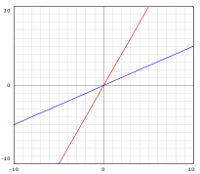# What does slope mean?

### Definition of slope:

The slope can be simply defined as the "steepness" of a line. A more advanced student might define it as the "rate of change" of a function, or "change in Y divided by change in X". If you're just learning the concept, think of it as a measure of how steep a line is.

### Example:

Here's a graph of the equation $$y = 2x$$ (red) and the equation $$y=0.5x$$ (blue). Note how the red line goes up 2 points for every 1 point it goes to the right. The blue line goes up 1/2 a point for every point it goes right.